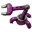## [1.1.50] create_spidertron() doesn't correctly scale lights

Post your bugs and problems so we can fix them.
Xorimuth
Filter InserterPosts: 430
Joined: Sat Mar 02, 2019 9:39 pm
Contact:

### [1.1.50] create_spidertron() doesn't correctly scale lights

Maybe this won't be considered a bug, as it is only encountered with mods and can be worked around with custom implementations, but it is vanilla code that is incorrect/incomplete.

Base mod provides a create_spidertron() function with optional arguments for scaling the spidertron. I've noticed that this doesn't fully scale the spidertrons lights so I've created some modifications to complete the scaling of these.

Here's what it looks like before and after applying my suggested fixes. Note that for these pictures I've removed the spidertron's ambient light (which is already scaled correctly) so that the incorrect eye_lights (as defined by light_positions) are emphasised.Screenshot 2021-12-31 at 18.50.46.png (470.22 KiB) Viewed 316 timesScreenshot 2021-12-31 at 18.52.24.png (734.34 KiB) Viewed 316 times
##### Before
spidertron_torso_graphics_set()

Code: Select all

``````  light =
{
{
minimum_darkness = 0.3,
intensity = 0.4,
size = 25 * spidertron_scale,
color = {r=1.0, g=1.0, b=1.0}
},
create_spidertron_light_cone(0,     1,    1   , -1),
create_spidertron_light_cone(-0.05, 0.7,  0.7  , 2.5),
create_spidertron_light_cone(0.04,  0.5,  0.45 , 5.5),
create_spidertron_light_cone(0.06,  0.6,  0.35 , 6.5)
},
light_positions = require("__base__/prototypes/entity/spidertron-light-positions"),
eye_light = {intensity = 1, size = 1, color = {r=1.0, g=1.0, b=1.0}},-- {r=1.0, g=0.0, b=0.0}},
``````
create_spidertron_light_cone()

Code: Select all

``````local function create_spidertron_light_cone(orientation, intensity, size, shift_adder)
local shift = { x = 0, y = -14 + shift_adder}
return
{
type = "oriented",
minimum_darkness = 0.3,
picture =
{
filename = "__core__/graphics/light-cone.png",
priority = "extra-high",
flags = { "light" },
scale = 2,
width = 200,
height = 200
},
source_orientation_offset = orientation,
shift = shift,
size = 2 * size,
intensity = 0.6 * intensity, --0.6
color = {r = 0.92, g = 0.77, b = 0.3}
}
end
``````
##### After
spidertron_torso_graphics_set()

Code: Select all

``````  light =
{
{
minimum_darkness = 0.3,
intensity = 0.4,
size = 25 * spidertron_scale,
color = {r=1.0, g=1.0, b=1.0}
},
create_spidertron_light_cone(0,     1,    1   , -1, spidertron_scale),
create_spidertron_light_cone(-0.05, 0.7,  0.7  , 2.5, spidertron_scale),
create_spidertron_light_cone(0.04,  0.5,  0.45 , 5.5, spidertron_scale),
create_spidertron_light_cone(0.06,  0.6,  0.35 , 6.5, spidertron_scale)
},
light_positions = scale_light_positions(require("__base__/prototypes/entity/spidertron-light-positions"), spidertron_scale),
eye_light = {intensity = 1, size = 1 * spidertron_scale, color = {r=1.0, g=1.0, b=1.0}},-- {r=1.0, g=0.0, b=0.0}},
``````
Using my custom function, which I'm sure could be improved:

Code: Select all

``````local function scale_light_positions(positions, scale)
local new_positions = {}
for index, position_list in pairs(positions) do
new_positions[index] = {}
for index2, position in pairs(position_list) do
local new_position = {position * scale, position * scale}
new_positions[index][index2] = new_position
end
end
return new_positions
end
``````
create_spidertron_light_cone()

Code: Select all

``````local function create_spidertron_light_cone(orientation, intensity, size, shift_adder, scale)
local shift = { x = 0, y = (-14 + shift_adder) * scale}
return
{
type = "oriented",
minimum_darkness = 0.3,
picture =
{
filename = "__core__/graphics/light-cone.png",
priority = "extra-high",
flags = { "light" },
scale = 2,
width = 200,
height = 200
},
source_orientation_offset = orientation,